# Eggs

3400 eggs sliced hens for February and March. We have to calculate how many hens can make 3400 eggs when one hen give 2 eggs a day for 59 days.

Correct result:

s =  28.8136

#### Solution:

3400 = s•2•59

118s = 3400

s = 170059 ≈ 28.813559

Calculated by our simple equation calculator.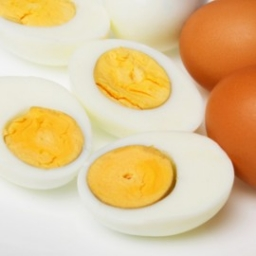We would be very happy if you find an error in the example, spelling mistakes, or inaccuracies, and please send it to us. We thank you!Tips to related online calculators
Do you have a linear equation or system of equations and looking for its solution? Or do you have quadratic equation?

## Next similar math problems:

• School marks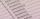Boris has a total of 22 marks. Ones have 3 times less than threes. There are two twos. How many has ones and threes?
• Chestnuts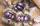Neil has 5-times chestnuts less than Adrian. Together they have 894 chestnuts. How many chestnuts has Neil and Adrian?
• FamilyThe twins Nina and Ema have five years younger brother Michal. Together have 43 years. How old is Michal?
• Jan and DanJan and Dan had the same money. Jan bought 5 workbooks and left him 15 CZK. Dan 6 and left him nothing. How much money have in total?
• Mystery numberA mystery number is between 800 and 920. No digits are the same. The difference of the numbers 5. What is the mystery number?
• Computer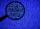A line of print on a computer contains 64 characters (letters, spacers or other chars). Find how many characters there are in 7 lines.
• SnacksThe school attends 344 pupils. Half of them take snacks. 13 pupils who took snacks did not attend school. How many snacks left?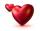Mom wants talk with dad 2.2 hours. But dad wants of 2 times more hours than mom ... How many hours wants dad talk with mom? ...
• Hotel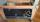The rooms in the mountain hotel are double and triple. Double rooms are 25 and triple are 17 more. How many rooms are there in this hotel?
• Apples 5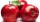In six crates are 45 kg of apples. In five crates were the same amounth and in one crate was 3 kg of apples more. How many kg of apples were in each crate?
• Apples 3Julka has 5 apples more than Hugo and four apples less than Annie. Hugo has 17 apples. How many apples has Julka and how Annie?
• Unknown number xyzFind the number that its triple is 24. Solve by equation.
• Carpenter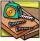You want to cut long board into smaller pieces. Carpenter said that one cut of the board into two pieces will cost you 70 cents. But you need to cut the board into 9 parts. How much you pay?
• Number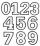Which number is 17 times larger than the number 6?
• MultiplesFind all multiples of 10 that are larger than 136 and smaller than 214.
• ProductResult of the product of the numbers 1, 2, 3, 1, 2, 0 is:
• Written numberPlace+values x ten thousands =30 thousands